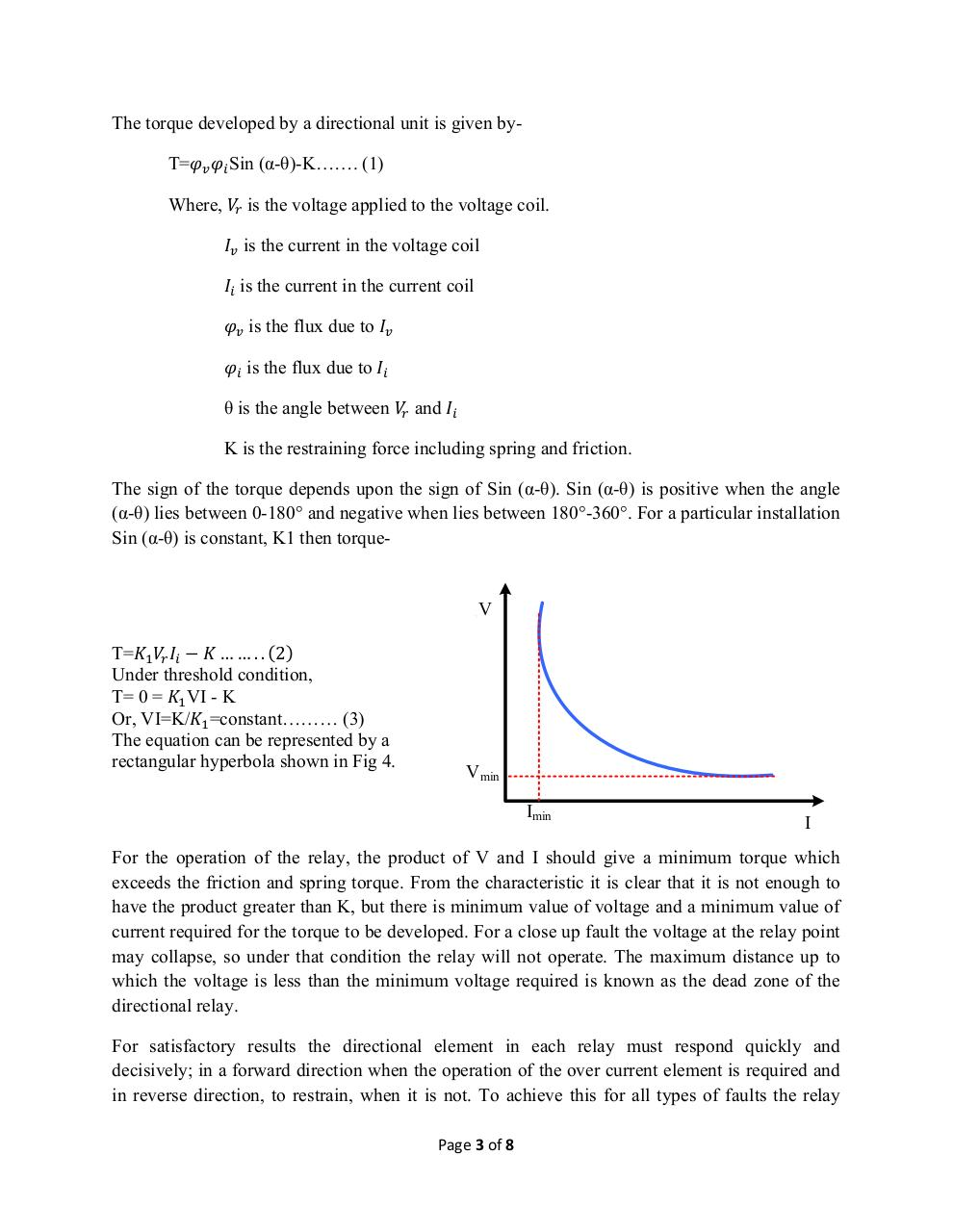PDF Archive

Easily share your PDF documents with your contacts, on the Web and Social Networks.

Directional ralys.pdfPage 1 2 3 4 5 6 7 8

Text preview

The torque developed by a directional unit is given byT=
Where,

Sin (α-θ)-K……. (1)
is the voltage applied to the voltage coil.
is the current in the voltage coil
is the current in the current coil
is the flux due to
is the flux due to
θ is the angle between

and

K is the restraining force including spring and friction.
The sign of the torque depends upon the sign of Sin (α-θ). Sin (α-θ) is positive when the angle
(α-θ) lies between 0-180° and negative when lies between 180°-360°. For a particular installation
Sin (α-θ) is constant, K1 then torque-

− …….. 2
T=
Under threshold condition,
T= 0 = VI - K
Or, VI=K/ =constant……… (3)
The equation can be represented by a
rectangular hyperbola shown in Fig 4.

V

Vmin
Imin

I

For the operation of the relay, the product of V and I should give a minimum torque which
exceeds the friction and spring torque. From the characteristic it is clear that it is not enough to
have the product greater than K, but there is minimum value of voltage and a minimum value of
current required for the torque to be developed. For a close up fault the voltage at the relay point
may collapse, so under that condition the relay will not operate. The maximum distance up to
which the voltage is less than the minimum voltage required is known as the dead zone of the
directional relay.
For satisfactory results the directional element in each relay must respond quickly and
decisively; in a forward direction when the operation of the over current element is required and
in reverse direction, to restrain, when it is not. To achieve this for all types of faults the relay
Page 3 of 8